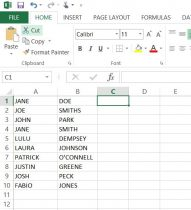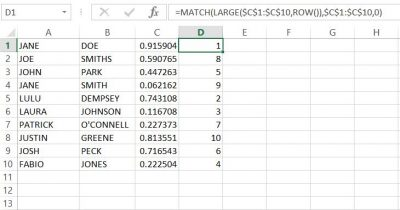## Microsoft Excel: Randomized Number I.D. for Participants

In order to present our data by the participant, the ethical thing was to avoid revealing the actual name of the individuals who gave us our data. In this, we used Microsoft Excel to generate and assign random numbers, rather than simply numbering every subject individually. These numbers would then act as the I.D.’s for each participant. On a separate spreadsheet, we put participants first and last names in columns ‘A’ and ‘B’ respectively (here I have put in ten fake names* to show you an example). For our data, we had a list of all the participants’ names in alphabetical order by last name.Next, I used the RAND, or random function. By putting the =RAND() function into column ‘C’ from cells C1 to C10, we were given a random decimal number. Then, I had tried to use the =RANDBETWEEN function in column ‘D’, inputting =RANDBETWEEN(1,10). Although this gave us a random whole number between 1 and 10, there were repeats of the same number. So now one of the biggest problems was finding a way to have excel create random intergers that did NOT repeat.Finally, with a little help from the library and the internet, I used the following formula to generate NON-REPEATING whole numbers in column ‘D’;
=MATCH(LARGE(\$C\$1:\$C\$20,ROW()),\$C\$1:\$C\$20,0)

The result was what we were looking for, anonymity for our participants. With this success, we copied and pasted the numbers next to the names in the list of participants in our data set.***None of these names are meant to have any relation to any person(s) alive or deceased.
**When I input the function into column ‘D’, the random values in column ‘C’ changed automatically, but remained random. you need to keep this formula in this column in order for the function in ‘D’ to work.

There may be other ways of achieving the same outcome, but this formula worked best in excel.